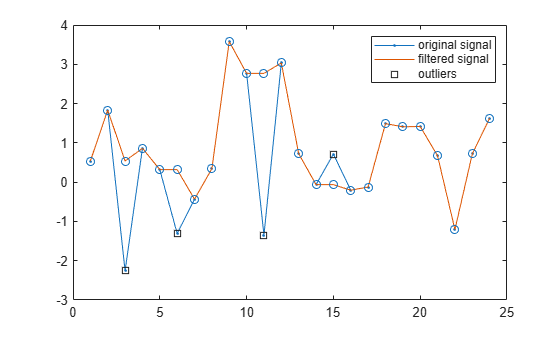Main Content

# Eliminate Outliers Using Hampel Identifier

This example shows a naive implementation of the procedure used by `hampel` to detect and remove outliers. The actual function is much faster.

Generate a random signal, `x`, containing 24 samples. Reset the random number generator for reproducible results.

```rng default lx = 24; x = randn(1,lx);```

Generate an observation window around each element of `x`. Take `k` = 2 neighbors at either side of the sample. The moving window that results has a length of $2×2+1=5$ samples.

```k = 2; iLo = (1:lx)-k; iHi = (1:lx)+k;```

Truncate the window so that the function computes medians of smaller segments as it reaches the signal edges.

```iLo(iLo<1) = 1; iHi(iHi>lx) = lx;```

Record the median of each surrounding window. Find the median of the absolute deviation of each element with respect to the window median.

```for j = 1:lx w = x(iLo(j):iHi(j)); medj = median(w); mmed(j) = medj; mmad(j) = median(abs(w-medj)); end```

Scale the median absolute deviation with

`$\frac{1}{\sqrt{2}\phantom{\rule{0.16666666666666666em}{0ex}}{erf}^{-1}\left(1/2\right)}\approx 1.4826$`

to obtain an estimate of the standard deviation of a normal distribution.

`sd = mmad/(erfinv(1/2)*sqrt(2));`

Find the samples that differ from the median by more than `nd` = 2 standard deviations. Replace each of those outliers by the value of the median of its surrounding window. This is the essence of the Hampel algorithm.

```nd = 2; ki = abs(x-mmed) > nd*sd; yu = x; yu(ki) = mmed(ki);```

Use the `hampel` function to compute the filtered signal and annotate the outliers. Overlay the filtered values computed in this example.

```hampel(x,k,nd) hold on plot(yu,'o','HandleVisibility','off') hold off```## Support

#### Deep Learning for Signal Processing with MATLAB

Download white paper